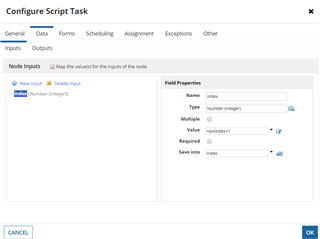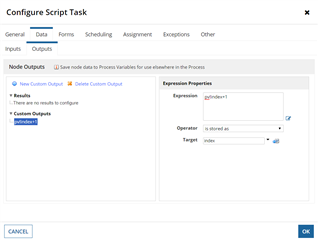# Evaluation order of inputs saveInto's and outputs in process model

We had a question pop up in our design review output the evaluation order of Data Input Save Input and Data Outputs. I know there is no guarantee on the order of Data Output execution, but I was wondering if there is a guarantee that Data Input Save Into's are evaluated first.

For example: Say before a script task, pv!index=0

And has the Data Inputs configured as belowAnd Data Outputs configured asWould the order evaluation be:

1. ac!index value = pv!index + 1 = 1
2. Save Into pv!index -> ac!index = 1
3. Outputs
• pv!index -> pv!index+1 = 2

So after execution: pv!index is guaranteed to be 2

Or could it potentially be:

1. ac!index value = pv!index + 1 =1
2. Outputs and Save Into's
• pv!index -> pv!index+1 = 1
• Save Into pv!index -> ac!index = 1

So after execution: pv!index could be 1 or 2 depending on the order of the Save Into's and Outputs were evaluated

A member of my team had heard that Save Into's and Outputs are evaluated together.

Parents